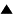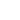# Metalanguage

In the scheme of control an important role is played by representation of the controlled system in the controlling device. When the controlled system consists of texts in some language L, the language used to make their representations in the controlling system -- let it be L' -- is referred to as a metalanguage. Creation of a metalanguage is a metasystem transition (MST), in which we ignore the controlling subsystem by abstraction. However, control is still there when the languages are used. Typically, it is a human being, both on the level of L and L'. The L' person uses the rules expressed in L', in order to guide and correct the manipulation of texts in L by the L person.

An example: algebraic notation is a metalanguage L' with respect to the language L of arithmetic. L includes a notation of numbers and the four arithmetic operations which can be executed by the L person. Representation of numbers in L' is the same as in L, but L' also includes letters as many-to-one representation of unspecified numbers. The algebraic person L' can do more than the arithmetic person L: he manipulates algebraic formulas and, in particular, knows how to solve equations. High school algebra is a metatheory with respect to arithmetic. But it is not the top of the hierarchy. Modern algebra is a metatheory with respect to high school algebra, as well as with respect to many other theories. For example, consider the following 3-level hierarchy. On the ground level (level 0) we have a finite set of symbols and strings of (this is comparable with countable objects of arithmetic). On the next level (control level 1) there is a device that performs various operations on strings: concatenation, permutation, etc. (compare it to arithmetic operations). Then on the level 2 we put group theory (which is a part of modern algebra) in its application to permutations on the level 1.

Apparently, David Hilbert was the first to use the prefix meta (from the Greek over) in the sense we use it in metalanguage, metatheory, and now metasystem. He introduced the term metamathematics to denote a mathematical theory of mathematical proof. In terms of our control scheme, Hilbert's MST has a non-trivial representation: a mapping of proofs in the form of usual mathematical texts (in a natural language with formulas) on the set of texts in a formal logical language which makes it possible to treat proofs as precisely defined mathematical objects. This done, the rest is as usual: the controlled system is a mathematician who proves theorems; the controlling person is a metamathematician who translates texts into the formal logical language and controls the work of the mathematician by checking the validity of his proofs and, possibly mechanically generating proofs in a computer. The emergence of the metamathematician is an MST.

We create a metalanguage and a metatheory in order to describe and examine some class of languages and theories. A metatheory M includes a representation of the examined theory T, and it can be seen as the perception in a control system where T is controlled by M. The result of the examination of the theories like T would, typically, be classification, modification and creation anew of the theories from the class to which T belongs. This is the action part of the control relation between T and M. This part, though, does not enter the meaning of the terms `metalanguage' and `metatheory': it may be absent. Hence we deal here with the case of a control system devoid of action.

 HomeMetasystem Transition TheoryCyberneticsControlSpecial Cases of Control / The Metasystem Transition Up Prev.Next Down